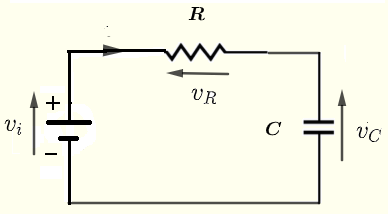# Low Pass RC Circuit Response to a Square Wave - Graphing Calculator

An online calculator to calculate and graph the voltage across a capacitor when the input $v_i(t)$ is a square wave.

## Formulae for Voltage in a Series RC Circuit to Square Wave

We first give the formulas of the voltage across the capacitor when the input is a square wave.The formulas developed in the study of low pass RC circuit response to a square wave is presented here its used in the calculator.
When a square wave of the form
$\displaystyle v_i(t) = V_0 \sum_{n=0}^{n=\infty} \left\{ u(t - n\;T)- u (t-(n+1/2)\;T) \right\}$ were $u(t)$ is the unit step function,
the voltage $v_C(t)$ across the capacitor is given by

$\displaystyle v_C(t) = \displaystyle V_0 \sum_{n=0}^{n=\infty} \left \{ u(t-nT) \; \left(1 - e^{- \dfrac{t - n \; T}{R \;C} } \right) \\\\ \quad \quad \quad - u(t-(n+1/2)T) \; \left(1 - e^{-\dfrac{ t - (n + 1/2) T}{\; R \; C} } \right) \right\}$
The voltage $v_R(t)$ across the resistor is given by
$v_R (t) = v_i(t) - v_C(t)$
The current $i(t)$ is given by
$i(t) = \dfrac{v_R}{R} = \dfrac{v_i(t) - v_C(t)}{R}$
$\tau = R C$ is called the time constant of the circuit.

## Use of the calculator

Enter the the resistance $R$, the capacitance $C$, the period $T$ of the square wave as a multiple of the time constant $\tau = R \; C$ and the number of periods to be displayed then press "Calculate&Graph".
The time constant $\tau$, the voltages $v_C(t)$ (blue) and $v_i(t)$ (red) are displayed.
The results shown are for $V_0 = 1$ V.

 Resistance R = 100 mΩ Ω KΩ MΩ Capacitance C = 100 pF nF μF mF F Period: T = 5 $\times$ RC Number of Periods to Display = 5

## Results

### More References and links

Low Pass RC Circuit Response to a Square Wave
series RLC circuit response to a step voltage
Engineering Mathematics with Examples and Solutions